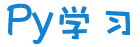注册    登录创作新主题

Python
 python开源   Django   Python   DjangoApp   pycharm
DATA
 docker   Elasticsearch

 问与答   闲聊   招聘   翻译   创业   分享发现   分享创造   求职   区块链   支付之战
aigc
 aigc   chatgpt
WEB开发
 linux   MongoDB   Redis   DATABASE   NGINX   其他Web框架   web工具   zookeeper   tornado   NoSql   Bootstrap   js   peewee   Git   bottle   IE   MQ   Jquery

Python88.com
 反馈   公告   社区推广

Py学习  »  Python

# Python金融分析系列-4：数学工具-近似、凸优化、积分和符号运算

👇 连享会 · 推文导航 | www.lianxh.cn

• 其一，希望大家经过四天的学习（尚需另外花费 1-2 个月演练吸收），能掌握基本的统计和计量分析方法，能理解多数期刊论文中使用的分析方法；

• 其二，希望诸位能建立起 Stata 的基本架构，熟知 Stata 能做什么、如何做？以便为后续学习打下宽厚扎实的基础。

The mathematicians are the priests of the modern world. ——Bill Gaede• 1. 缘起

• 2. 近似

• 2.1 回归

• 2.2 定制化基准函数

• 3. 凸优化

• 3.1 全局最优化

• 3.2 局部最优化

• 3.3 受限约束

• 4. 符号运算

• 4.1 积分

• 4.2. 微分

• 5. 小结

• 6. 相关推文

• 近似
• 凸优化
• 积分
• 符号运算

## 2. 近似

import numpy as npfrom pylab import plt, mplplt.style.use('seaborn')mpl.rcParams['font.family'] = 'serif'%matplotlib inline

def f(x):    return np.sin(x)+0.5*x

# plot the function over a fixed intervaldef create_plot(x, y, styles, labels, axlabels):    plt.figure(figsize=(10,6))    for i in range(len(x)):        plt.plot(x[i], y[i], styles[i], label=labels[i])    plt.xlabel(axlabels)    plt.ylabel(axlabels)    plt.legend(loc=0)
x = np.linspace(-2*np.pi, 2*np.pi, 50)create_plot([x], [f(x)], ['b'],['f(x)'], ['x', 'f(x)'])

### 2.1 回归



res = np.polyfit(x, f(x), deg=1, full=True, cov=True)
(array([ 4.28841952e-01, -1.33457859e-16]),array([21.03238686]),2,array([1., 1.]),1.1102230246251565e-14)
ry = np.polyval(res, x)
create_plot([x, x], [f(x), ry], ['b', 'r.'],            ['f(x)', 'regression'], ['x', 'f(x)'])

res5 = np.polyfit(x, f(x), deg=5)ry5 = np.polyval(res5, x)create_plot([x, x], [f(x), ry5], ['b', 'r.'],            ['f(x)', 'regression'], ['x', 'f(x)'])

### 2.2 定制化基准函数

matrix = np.zeros((3+1, len(x)))matrix[0,:] = 1matrix[1,:] = xmatrix[2,:] = x ** 2matrix[3,:] = x ** 3reg = np.linalg.lstsq(matrix.T, f(x), rcond=None)ry = np.dot(reg, matrix)create_plot([x, x], [f(x), ry], ['b', 'r.'],            ['f(x)', 'regression'], ['x', 'f(x)'])

matrix[3,:] = np.sin(x)reg = np.linalg.lstsq(matrix.T, f(x), rcond=None)ry = reg@matrixcreate_plot([x, x], [f(x), ry], ['b', 'r.'],            ['f(x)', 'regression'], ['x', 'f(x)'])

xu = np.random.rand(50) *4 *np.pi - 2*np.piyu = f(xu)reg = np.polyfit(xu, yu, deg=5)ry = np.polyval(reg, xu)create_plot([xu, xu], [yu, ry], ['b.', 'ro'],['f(x)', 'regression'], ['x', 'f(x)'])

## 3. 凸优化

def fm(p):    x, y = p    return (np.sin(x) + 0.05*x**2            + np.sin(y)+0.05*y**2)

x = np.linspace(-10,10,50)y = np.linspace(-10,10,50)X, Y = np.meshgrid(x, y)Z = fm((X, Y))fig = plt.figure(figsize=(15, 6))ax = fig.add_subplot(projection='3d')surf = ax.plot_surface(X, Y, Z, rstride=2, cstride=2,cmap='coolwarm', linewidth=0.5,antialiased=True)ax.set_xlabel('x')ax.set_ylabel('y')ax.set_zlabel('f(x, y)')fig.colorbar(surf, shrink=0.5, aspect=5)

### 3.1 全局最优化

import scipy.optimize as sco

def fo(p):    x, y = p    z = np.sin(x) + .05 * x**2 + np.sin(y) +0.05*y**2    if output == True:        print(

f"{x} | {y} | {z}")    return z
output = Truesco.brute(fo, ((-10, 10.1, 5), (-10, 10.1, 5)), finish=None)

output = Truesco.brute(fo, ((-10,10.1,0.1),(-10,10.1,0.1)), finish=None)

### 3.2 局部最优化

output = Trueopt3 = sco.fmin(fo, opt2, maxiter=15)

output = Falsesco.fmin(fo, (2.0, 2.0), maxiter=250)

### 3.3 受限约束

def EU(p):    s, b = p    return -(0.5*np.sqrt(s*15 + b*5)+             0.5*np.sqrt(s*5 + b*12))cons = ({'type':'ineq',         'fun': lambda p: 100 - p*10 - p*10})bnds = ((0, 1000),(0, 1000))result = sco.minimize(EU, [5,5], method="SLSQP",                      bounds=bnds, constraints=cons)
 message: Optimization terminated successfully success: True  status: 0     fun: -9.700883611487832       x: [ 8.025e+00  1.975e+00]     nit: 5     jac: [-4.851e-01 -4.849e-01]    nfev: 16    njev: 5

## 4. 符号运算

sympy 中的一个基本类是 Symbol

x = sy.Symbol('x')y = sy.Symbol('y')f = x**2 +3+0.5*x**2 + 3/2sy.simplify(f)

sy.solve(x**2 -1)sy.solve(x**2-1-3)sy.solve(x**3 +0.5*x**2 -1)

### 4.1 积分

sympy 另外一个重要的优势在于其积分和微分的功能:

a,b = sy.symbols('a,b')
sy.Integral(sy.sin(x) + 0.5*x, (x, a,b))sy.integrate(sy.sin(x) + 0.5*x, x)

sy.integrate(sy.sin(x) + 0.5*x, (x, 0.5, 9.5))

### 4.2. 微分

int_fun = -sy.cos(x)+0.25*x**2int_fun.diff()

# example of an equation with interactive x and yf = (sy.sin(x) + 0.5*x*y - sy.cos(y) - 0.6*y**2)

del_x = sy.diff(f,x)del_y = sy.diff(f,y)
x0,y0 = sy.nsolve((del_x, del_y), (x,y), (-1,1))f.subs({x:x0, y:y0}).evalf()

## 6. 相关推文

Note：产生如下推文列表的 Stata 命令为：
lianxh python

ssc install lianxh, replace

• 专题：Python-R-Matlab
• 初虹, 2022, Python爬虫1：小白系列之requests和json, 连享会 No.887.
• 初虹, 2022, Python爬虫2：小白系列之requests和lxml, 连享会 No.888.
• 周豪波, 2020, Python 调用 API 爬取百度 POI 数据小贴士——坐标转换、数据清洗与 ArcGIS 可视化, 连享会 No.20.
• 周豪波, 2020, Python 调用 API 进行逆地理编码, 连享会 No.59.
• 孔亦泽, 2023, 分享：公开数据库 及 数据格式转换-R-Stata-Python, 连享会 No.1272.
• 孙斯嘉, 2020, Python 调用 API 爬取百度 POI 数据, 连享会 No.60.
• 孙斯嘉, 2020, Python 调用 API 进行地理编码, 连享会 No.62.
• 左从江, 2020, Python: 批量爬取下载中国知网(CNKI) PDF论文, 连享会 No.54.
• 张春雷, 2022, Stata+Python：导入超大Excel文档的新思路-以国泰安为例, 连享会 No.1021.
• 张洪洋, 2023, Python：文本数据增强, 连享会 No.1240.
• 张翠燕, 2020, Python+Wind：用 Pyautogui 轻松下载 Wind 数据, 连享会 No.257.
• 杨晓军, 2022, Stata-Python交互-10：Stata17 新特性之PyStata的配置与应用, 连享会 No.996.
• 梁淑珍, 2022, Python：Jaccard 相似度和距离, 连享会 No.1043.
• 梁淑珍, 2022, Python：文本分析必备—搜狗词库, 连享会 No.1078.
• 王俊, 2020, Python+Stata：批量制作个性化结业证书, 连享会 No.236.
• 王卓, 2022, Python文本分析：将词转换为向量-Word2Vec, 连享会 No.1134.
• 王卓, 2023, Python：从随机实验到双重机器学习, 连享会 No.1204.
• 王卓, 2022, Python：绘制动态地图-pyecharts, 连享会 No.922.
• 王颖, 2022, Python爬取静态网站：以历史天气为例, 连享会 No.921.
• 王颖, 2022, Python：多进程、多线程及其爬虫应用, 连享会 No.935.
• 王颖, 2022, Python：爬取动态网站, 连享会 No.932.
• 秦利宾, 2020, Python：爬取上市公司公告-Wind-CSMAR, 连享会 No.131.
• 秦利宾, 2021, Python：爬取巨潮网公告, 连享会 No.646.
• 范思妤, 2023, Python：基于selenium爬取科创板审核问询, 连享会 No.1172.
• 许梦洁, 2020, Python: 6 小时爬完上交所和深交所的年报问询函, 连享会 No.104.
• 许梦洁, 2020, Python: 使用正则表达式从文本中定位并提取想要的内容, 连享会 No.91.
• 许梦洁, 2020, Python: 如何优雅地管理微信数据库？, 连享会 No.90.
• 许梦洁, 2021, Python爬虫：从SEC-EDGAR爬取股东治理数据-Shareholder-Activism, 连享会 No.744.
• 许梦洁, 2021, Python爬虫：爬取华尔街日报的全部历史文章并翻译, 连享会 No.743.
• 许梦洁, 2020, Python：拆分文件让百万级数据运行速度提高135倍, 连享会 No.301.
• 连享会, 2021, 司继春：Python学习建议和资源, 连享会 No.563.
• 郭李鹏, 2020, Stata交互：Python-与-Stata-对比, 连享会 No.303.
• 专题：Stata入门
• 秦利宾, 2020, Stata 与 Jupyter Notebook 关联的两种方法, 连享会 No.136.
• 许梦洁, 2020, 使用 Jupyter Notebook 配置 Stata\Python\Julia\R, 连享会 No.84.
• 韩少真, 展金永, 2020, 珠联璧合 II：Jupyter Notebook 与 Matlab 之融合, 连享会 No.116.
• 专题：Stata命令
• 刘欣妍, 2022, Stata与Python等价命令, 连享会 No.955.
• 专题：Stata教程
• 连享会, 2021, Stata-Python交互-1：二者配合的基本设定, 连享会 No.551.
• 连享会, 2021, Stata-Python交互-2：在Stata中调用Python的三种方式, 连享会 No.552.
• 连享会, 2021, Stata-Python交互-3：如何安装Python宏包, 连享会 No.553.
• 连享会, 2021, Stata-Python交互-4：如何调用Python宏包, 连享会 No.554.
• 连享会, 2021, Stata-Python交互-5：边际效应三维立体图示, 连享会 No.555.
• 连享会, 2021, Stata-Python交互-6：调用APIs和JSON数据, 连享会 No.556.
• 连享会, 2021, Stata-Python交互-7：在Stata中实现机器学习-支持向量机, 连享会 No.557.
• 连享会, 2021, Stata-Python交互-8：将Stata数据导入Python, 连享会 No.558.
• 连享会, 2021, Stata-Python交互-9：将python数据导入Stata, 连享会 No.559.
• 专题：Stata程序
• 连玉君, 2020, Stata程序：是否有类似-Python-中的-zip()-函数, 连享会 No.347.
• 专题：其它
• 田原, 2020, ES 期望损失: Stata 及 Python 实现, 连享会 No.182.
• 专题：数据分享
• 秦利宾, 许梦洁, 2021, Python+Stata：如何获取中国气象历史数据, 连享会 No.793.

🍓 课程主页https://www.lianxh.cn

New！ Stata 搜索神器：lianxhsongbl  GIF 动图介绍

👉 安装：
. ssc install lianxh
. ssc install songbl
👉  使用：
. lianxh DID 倍分法
. songbl all

#### 🍏 关于我们

• 连享会 ( www.lianxh.cn，推文列表) 由中山大学连玉君老师团队创办，定期分享实证分析经验。
• 直通车： 👉【百度一下： 连享会】即可直达连享会主页。亦可进一步添加 「知乎」,「b 站」,「面板数据」,「公开课」 等关键词细化搜索。

Python社区是高质量的Python/Django开发社区